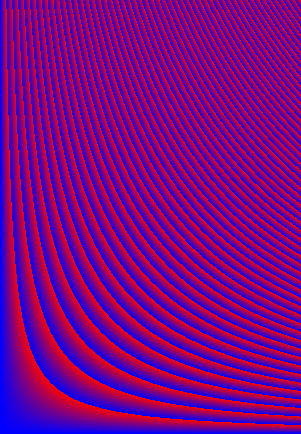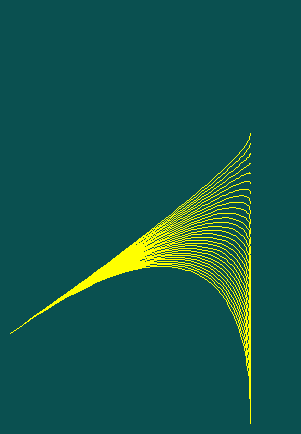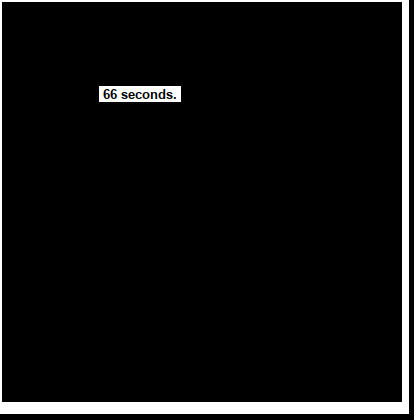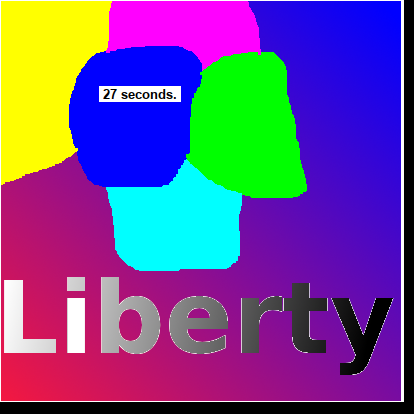# Advantages of using an array to store an image and manipulate itexample images## Why do this?

LB has had no getpixel function, and drawing pixel by pixel is also slow- an inevitable penalty of how images are stored and manipulated in Windows.

My first HOME computing experience was with early BASIC on RCA1802 chips and then on a Commodore PET. We could PEEK and POKE any location in our meagre RAM storage. A section, say 320x240, was also readable every say 1/50 sec by a display chip and sent to the screen modulater and hence to a TV. The microprocesser ran at say 1 MHz. The BASIC interpreter could set points on the screen quite fast, despite being 18 bit and 5000 times slower than present day machines. The screen refresh took exactly the same time whether pixels had been changed or not. Screen was simple memory- read and write it as fast as you can...

Particular fun could be had by pointing the display chip to a different address for use as screen display. Low memory had interesting things going on with keyboard buffers and timer which became visible on screen as they changed... And there was no protection of memory areas- you could crash a running machine easily by POKEing important memory locations.

As machine architecture advanced and speeds increased, we got more sophisticated situations- not necessarily to the advantage of coding languages. Nowadays, when you set a pixel it is changed in an area that will be later read and displayed- but ALL of an array has to be read and displayed at every screen refresh, even if only one pixel has changed. And reading/writing pixels is slowed by this, and also by having such a big range of colours.

## Setting pixels in a graphic.

Look how long it takes to set all pixels of a 400x400 image to black, one at a time!```    nomainwin

WindowWidth  =420
WindowHeight =444

open "Slow 'set' of pixels" for graphics_nsb as #wg

#wg "trapclose [quit]"

#wg "down"

now =time\$( "seconds")

for y =1 to 400
for x =1 to 400
#wg "set "; 2 +x; " "; 2 +y
scan
next x
next y

#wg "up ; goto 100 100 ; down"
#wg "backcolor white ; color black"
#wg "\ "; str\$( time\$( "seconds") -now); " seconds. "
#wg "getbmp scr 1 1 420 420"
bmpsave "scr", "LBsetPixel.bmp"

wait

[quit]
close #wg
end

```

## Reading pixels from a graphic in native LB code. Method 1

Look how long it takes to read all pixels of a 400x400 image! Here I use the method of saving each pixel to a file and reading the BGR components' this method works in LB and in JB.```    'nomainwin

WindowWidth  =415
WindowHeight =432

open "Slow 'read' of pixels with native LB" for graphics_nsb as #wg

#wg "trapclose [quit]"

#wg "down"

#wg "drawbmp scr 2 2"
#wg "flush"

now =time\$( "seconds")

for y =1 to 400
print y
for x =1 to 400
dummy\$  =getPixel\$( x, y)
scan
next x
next y

#wg "up ; goto 100 100 ; down"
#wg "backcolor white ; color black"
#wg "\ "; str\$( time\$( "seconds") -now); " seconds. "
#wg "getbmp scr 1 1 420 420"
bmpsave "scr", "LBnativeGetPixel.bmp"

wait

function getPixel\$( x, y)
#wg "getbmp scr "; x; " "; y; " 1 1"
bmpsave "scr", "scr.bmp"

open "scr.bmp" for input as #fIn
contents\$ =input\$( #fIn, lof( #fIn))
blue  =asc( mid\$( contents\$, 67, 1))
green =asc( mid\$( contents\$, 68, 1))
red   =asc( mid\$( contents\$, 69, 1))
close #fIn

getPixel\$ =str\$( blue) +" " +str\$( green) +" " +str\$( red)

end function

[quit]
close #wg
end
```

## Method indexing into loaded image info.

This method loads the whole source BMP into memory as an array, and indexes into it to read the pixel colour triads. It writes output images from the array.

The method works with BMP files, but there are about 8 variations on these, and you have to parse the preface section to locate the data, and be aware that image widths other than three introduce padding bytes that you have to allow for. This is perfectly do-able BUT it is easier to arrange for source files to have widths which are a multiple of four, and are saved as 24 bit without colour information! Load them in your painting application- ( GIMP for me) and re-save as a BMP file, 24 bit.

However, MUCH easier is the ppm P6 format. This has all the pixel data as consecutive rows of colour triads. You just jump to this past a few preamble bytes- done with a single 'word\$()' line!code

## LB's method, calling a dll.

This method relies on LB's ability to call a dll to read an image's pixel values. It therefore can't be used in JB.```    'nomainwin

WindowWidth  =415
WindowHeight =432

open "Slow 'read' of pixels with native LB" for graphics_nsb as #wg

handle =hwnd( #wg)
calldll #user32, "GetDC", handle as ulong, hDC as ulong
#wg "trapclose [quit]"

#wg "down"

#wg "drawbmp scr 2 2"
#wg "flush"

now =time\$( "seconds")

for y =1 to 400
print y
for x =1 to 400
dummy\$  =getPixel\$( x, y)
scan
next x
next y

#wg "up ; goto 100 100 ; down"
#wg "backcolor white ; color black"
#wg "\ "; str\$( time\$( "seconds") -now); " seconds. "
#wg "getbmp scr 1 1 420 420"
bmpsave "scr", "LBdllGetPixel.bmp"

wait

function getPixel\$( x, y)
calldll #gdi32, "GetPixel", hDC as ulong, v as long, yy as long,  pixcol as long

blue  = int(  pixcol /( 256*256))
green = int( (pixcol               -bl *256*256) / 256)
red   = int(  pixcol               -bl *256*256 - gr *256)

getPixel\$ =str\$( blue) +" " +str\$( green) +" " +str\$( red)
end function

[quit]
callDll #user32, "ReleaseDC", h as ulong, hDC as ulong, result as ushort
close #wg
end
```

## The array method

By storing pixel data as a two-dimensional array of RGB triads, you can read/write pixel by pixel very quickly. Faster than calling a dll or saving and reading one-pixel files! You need your own routines to replace set, line and circle, but now have fast routines do do their job, and a fast getpixel as a bonus. You can also save easily to bmp or ppm.

This is also the basis of a quicker method of reading pixels- treat the whole image as a file and index into it to find a pixel's BGR values.

By implementing Breseham's technique to fit lines between points, it becomes easy also to fit quadratic and cubic Bezier curves. This enabled me to write solutions to half a dozen Rosetta Code tasks.

The method comes into its own if you want to step beyond setting points and straight lines and circles, especially if you want very large images..

I really need now to add a fill routine! Doing fills in LB is painfully slow- you really HAVE to use the dll method. And fills need you to know a point to start from that is inside your chosen figure...

## Code

I was able to take a BMP image and swap R and B components, or replace RBG with GreyGreyGrey, or 'vignette' all within a circle in seconds. Just writing pixels with 'set' takes MUCH longer.Loading the first, pre-drawn image, and producing two variants now takes only seconds..

The following code is an example from when I was developing these ideas. Will replace with a zip file of some more streamlined examples...

```     '  *************************************************************************
'  **                                                                     **
'  **       new10.bas           Nov 28 2020          tenochtitlanuk       **
'  **                                                                     **
'  **   Makes various computer-generated figures as bmp and as ppm files. **
'  **                                                                     **
'  *************************************************************************
'
'
'  To-dos...
'      Add readBmp sub to read a 24 bit bmp into the array and set Width nd Height
'      Add swapRGB sub to swap order of r g and b
'      Add toGreyscale to change to a b&w brightness image
'      Add routines like vignette, swirl, waver...
'
'
'
nomainwin
'
global Width, Height, CR\$, pi, DS\$
'
Width     =402    '   so Width +1 pixels
Height    =433    '   by Height+1 pixels
'
CR\$       =chr\$( 10)
pi        =4 *atn( 1)
'
dim Pix\$( Width +1, Height +1)                          '   set up local pixel arrays Pix\$( x, y) holding 'RGB' as 3 bytes)
dim Pix( 2000), Piy( 2000)                              '   to hold values in subs
'
'
for y =0 to Height
for x =0 to Width
p   =int( (x *y /10) mod 255)
Pix\$( x, y) =chr\$(  p) +chr\$( 0) +chr\$( 255 -p)   '   in R G B order
next x
next y
'
'
call fill 255, 255,  80
for K =250 to 40 step -5
call bresenham 10, 100,   250, K,     0, 0, 255
next K
call savePpm "BresenhamLines"
call saveBmp "BresenhamLines"
'
'
call fill 255,  80, 255
for RR =10 to 150 step 10
call circle 200, 200, RR, 0, 255, 0
next RR
call savePpm "circles"
call saveBmp "circles"
'
'
call fill 0, 0, 200
Ra      = 60
for theta =0 to 4 *pi step 0.0003
Xc      =int( radius *sin( theta) +300.5)
Yc      =int( radius *cos( theta) +150.5)
radius  =Ra *( 2 +sin( theta *5.1))
Ra      =Ra *0.999999
call setpixel Xc, Yc, 0, 255, 255
next theta
call savePpm "wavyCircles"
call saveBmp "wavyCircles"
'
'
call fill 10, 80, 80
for y =10 to 300 step 10
call bezierquad 10, 100,  250, 270,  250, y,   40,    255, 255, 255
next y
'
'
call fill 10, 189, 80
for y =10 to 300 step 10
call beziercubic  160, 250,    200, y,      230,   100,      20, 10,     60
next y
call savePpm "BezierCubic"
call saveBmp "BezierCubic"
wait'
'
'
'call histogramAndBandW   '   N/A
'''call savePpm "Pf"
'notice "Done!"
wait
'
'   ________________________________________________________________________
sub fill r, g, b
for y =0 to Height
for x =0 to Width
Pix\$( x, y) =chr\$( r) +chr\$( g) +chr\$( b)
next x
next y
end sub
'
'
sub setpixel x, y,   r, g, b
if x >=0 and x <=Width and y >=0 and y <=Height then Pix\$( x, y) =chr\$( r) +chr\$( g) +chr\$( b)
end sub
'
'
sub savePpm n\$
'Save as a ppm file . . .
print "Saving "; n\$; ".ppm"
open n\$ +".ppm" for output as #fOut
print #fOut, "P6"                                       +CR\$;
print #fOut, "# Created by Liberty BASIC"               +CR\$;
print #fOut, str\$( Width +1)  +" " +str\$( Height +1)    +CR\$;
print #fOut, "255"                                      +CR\$;
for y =Height to 0 step -1
for x =0 to Width
print #fOut, Pix\$( x, y);   '   ie in R G B  order..
next x
next y
close #fOut
end sub
'
'
sub saveBmp fn\$                        '    needs global Width, Height and array of pixels Pix\$( Width +1, Height +1)
w           =Width  +1
h           =Height +1

open fn\$ +".bmp" for output as #bmp

filetype\$   ="BM"
reserved\$   =FourByteString\$(  0)
offset\$     =FourByteString\$( 54)
width\$      =FourByteString\$( w)
height\$     =FourByteString\$( h)
last        =( w *3) mod 4                  '   find how many of the last 4 bytes are already used
filesize    =54 +( w +pad) *3 *h       '   ie header ( 54 bytes) +bitmapdata ( calculated).
filesize\$   =FourByteString\$( filesize)
#bmp filetype\$ +filesize\$ +reserved\$ +offset\$;

planes\$     =chr\$(  1) +chr\$( 0)
bits\$       =chr\$( 24) +chr\$( 0)                '   data stored in triple-bytes, 8 bits per colour.
compression\$=FourByteString\$( 0)
ignore\$     =FourByteString\$( 0) +FourByteString\$( 0) +FourByteString\$( 0) +FourByteString\$( 0)
bmpsize\$    =FourByteString\$( filesize)
#bmp bmpheader\$ +width\$ +height\$ +planes\$ +bits\$ +compression\$ +bmpsize\$ +ignore\$;  '   <<<<<<

for y =0 to Height
bytes   =0
for x =0 to Width                     '   data is  B G R  pixels.
#bmp right\$( Pix\$( x, y), 1) +mid\$( Pix\$(  x, y), 2, 1) +left\$( Pix\$( x, y), 1);                                          '   <<<<<<
bytes   =bytes +3
next x
'   however each row has to be represented by multiples of four bytes, so add as necessary.
if ( bytes mod 4) <>0 then b =4 -( bytes mod 4)
for k =1 to b
#bmp chr\$( 0);  '   could be any byte as padding....                               '   <<<<<<
bytes   =bytes +1
next k
next y
close #bmp
end sub

function rev\$( i\$)
rev\$ =mid\$( i\$, 3, 1) +mid\$( i\$, 2, 1) +mid\$( i\$, 1, 1)
end function
function sgn( x)
if x >=0 then sgn =1 else sgn =0 -1
end function
'   ________________________________________________________________________
sub circle cx, cy,    rd,    r, g, b
'LOCAL f, x, y, ddx, ddy
f     =1 -rd
y     =rd
ddy   =0 -2 *rd
call setpixel cx,    cy +rd, r, g, b
call setpixel cx,    cy -rd, r, g, b
call setpixel cx +rd, cy,    r, g, b
call setpixel cx -rd, cy,    r, g, b
while x =0 then
y     = y -1
ddy   =ddy +2
f     =f +ddy
end if
x       =x       +1
ddx     =ddx     +2
f       =f + ddx +1
call setpixel cx +x, cy +y,   r, g, b
call setpixel cx -x, cy +y,   r, g, b
call setpixel cx +x, cy -y,   r, g, b
call setpixel cx -x, cy -y,   r, g, b
call setpixel cx +y, cy +x,   r, g, b
call setpixel cx -y, cy +x,   r, g, b
call setpixel cx +y, cy -x,   r, g, b
call setpixel cx -y, cy -x,   r, g, b
wend
end sub
sub bresenham x1, y1,   x2, y2,   r, g, b
dx =abs( x2 -x1): sx =sgn( x2 -x1)
dy =abs( y2 -y1): sy =sgn( y2 -y1)
if dx dy then
x1 =x1 +sx: e =e -dy: if e <0 then e =e +dx: y1 =y1 +sy
else
y1 =y1 +sy: e =e -dx: if e <0 then e =e +dy: x1 =x1 +sx
end if
loop until 0
end sub
'   _________________________________________________________
sub bezierquad x1,y1,  x2,y2,  x3,y3, n,  r, g, b
for i = 0 to n
t       =i /n
t1      =1 -t
a       =t1^2
b       =2 *t *t1
c       =t^2
Pix( i) =int( a *x1 +b *x2 +c *x3 +0.5)
Piy( i) =int( a *y1 +b *y2 +c *y3 +0.5)
next i
for i =0 to n -1
call bresenham Pix( i), Piy( i),   Pix( i +1), Piy( i +1),    r, g, b
next i
end sub
'   ________
sub beziercubic x1, y1,    x2, y2,    x3, y3,    x4, y4,    n
for i = 0 to n
t       =i /n
t1      =1 -t
a       =t1^3
b       =3 *t *t1^2
c       =3 *t^2 *t1
d       =t^3
Pix( i)  =int( a *x1 +b *x2 +c *x3 +d *x4 +0.5)
Piy( i)  =int( a *y1 +b *y2 +c *y3 +d *y4 +0.5)
next i
for i =0 to n -1
call bresenham Pix( i), Piy( i),   Pix( i +1), Piy( i +1),    r, g, b
next i
end sub
'   ______<<<<<<< bring back as a sub or function

' Create a ppm version of an existing bmp
call fill 0, 0, 0 '   initialise all pixels to black...
open "a.bmp" for input as #fIn
da\$         =input\$( #fIn, 53) '   get past bmp preamble 53 bytes
for y =0 to Height step 1
for x =0 to Width
da\$         =input\$( #fIn, 3)
Pix\$( x, y) =rev\$( da\$) '    rgb --> bgr
if x mod 501 =0 then da\$ =input\$( #fIn, 1) ' get past the padding byte
next x
next y
close #fIn
''call savePpm "lazarus3"

function FourByteString\$( i)
b1  = i mod 2^8
b1\$ =chr\$( b1)
i   =( i -b1) /2^8
if i <0 then i =0

b2  = i mod 2^8
b2\$ =chr\$( b2)
i   =( i -b2) /2^8
if i<0 then i =0

b3 = i mod 2^8
b3\$ =chr\$( b3)
i   =( i -b3) /2^8
if i<0 then i =0

b4 = i mod 2^8
b4\$ =chr\$( b4)
FourByteString\$ = b1\$ +b2\$ +b3\$ +b4\$
end function

```